## Topic: Counting

Free Printable Worksheets on Counting

## Basic Statistics: Probability I (Set 4)Worksheet on basic probability – The student is asked several questions about the number of shapes in the box and to find the probabilities of selecting different shapes at random....

## Counting Chart 1 to 100 (blank)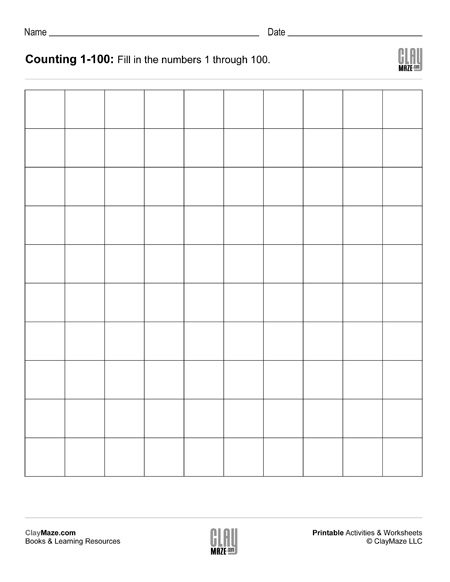Free and printable blank number chart for the numbers 1 to 100....

## Counting Chart 1 to 100 (set 1)1 to 100 number chart worksheet for kindergarten and first grade. In this worksheet the student is asked to fill in the missing numbers from 1 to 100. Half of them are filled in....

## Counting Chart 1 to 100 (set 2)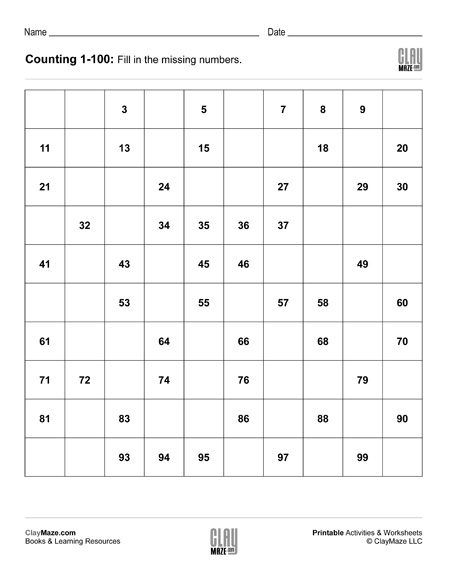Kindergarten and first grade counting worksheet for the numbers 1 through 100. Half of the numbers are missing in the chart and the student is asked to fill in those missing numbers....

## Counting Chart 1 to 100 (set 3)Kindergarten and elementary school number chart worksheet for the numbers 1 to 100. The student is asked to fill in the missing number counting by 1’s....

## Counting Chart 1 to 100 (set 4)Counting worksheet: Numbers 1 to 100. Fill in the missing numbers. Free and printable number chart worksheet....

## Skip Counting Worksheet – Counting by 2’s and Counting by 5’s (blank chart)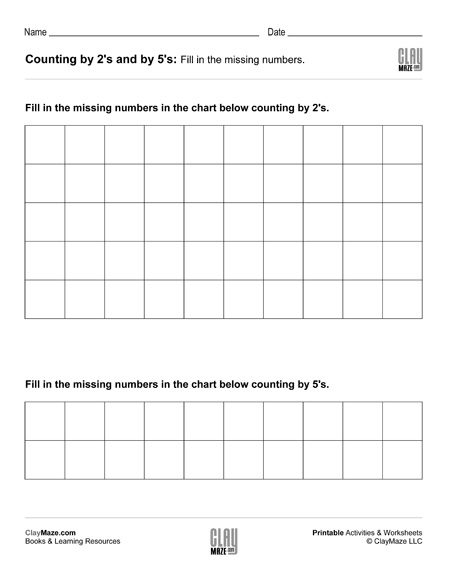Free and printable blank skip counting number chart for the numbers up to 100....

## Skip Counting Worksheet – Counting by 2’s and Counting by 5’s (Set 1)Up 100 skip counting number charts worksheet. The student is asked to fill in the missing numbers up to 100 counting by 2’s and counting by 5’s....

## Skip Counting Worksheet – Counting by 2’s and Counting by 5’s (Set 2)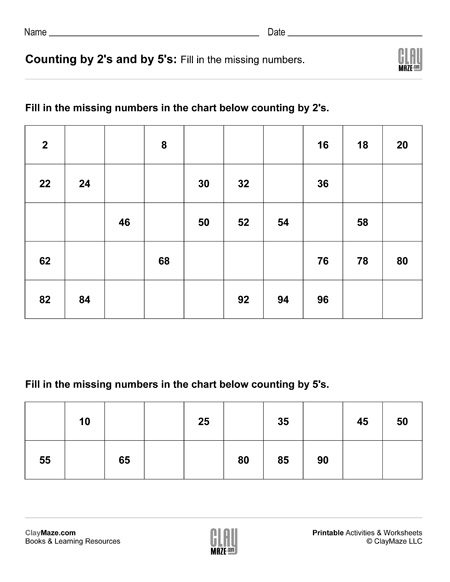Skip counting worksheet for numbers up to 100. Count by 2’s to fill in the first chart and count by 5’s to fill in the second....

## Skip Counting Worksheet – Counting by 2’s and Counting by 5’s (Set 3)Skip counting worksheet with 2 charts. Counting by 5’s and counting by 2’s....

## Skip Counting Worksheet – Counting by 2’s and Counting by 5’s (Set 4)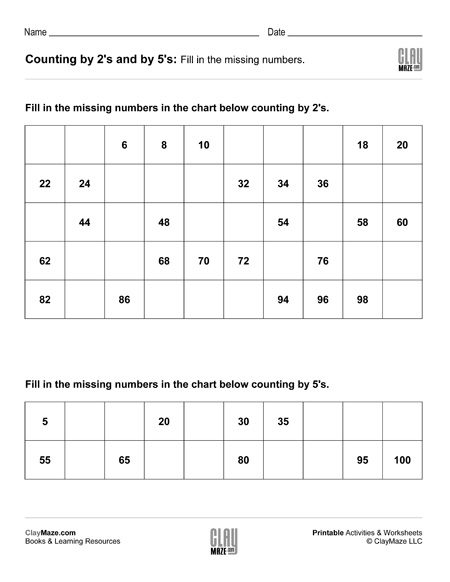Skip counting worksheet – Numbers up to 100. Fill in the missing numbers counting by 2’s and counting by 5’s....